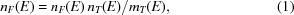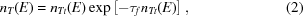## letters to the editorJOURNAL OFSYNCHROTRONRADIATION
ISSN: 1600-5775

## Comments on Treatment of EXAFS data taken in the fluorescence mode in non-linear conditions by G. Ciatto et al. (2004). J. Synchrotron Rad.11, 278–283

aX-ray Instrumentation Associates, 8450 Central Avenue, Newark, CA 94560, USA
*Correspondence e-mail: bill@xia.com

(Received 1 September 2004; accepted 30 September 2004)

We applaud the recent efforts of Ciatto, d'Acapito, Boscherini & Mobilio [J. Synchrotron Rad. (2004), 11, 278–283] to extend the useful operating range of EXAFS data collection as presented in their recent article Treatment of EXAFS data taken in the fluorescence mode in non-linear conditions. Methods that work accurately at ever higher input counting rates not only produce more science in less time but also allow synchrotron sources to be used more effectively. In support of these goals, we would like to suggest that, not only can the accuracy of their method II be further increased, but that it should also have a much wider range of applicability than the authors indicate.

In method II, the measured number of counts mF(E) in the fluorescence window at each measured energy E in the EXAFS spectrum is corrected to obtain the true' number of counts nF(E) that would have been measured in the absence of pile-up according towhere mT(E) is the total number of counts placed into the complete spectrum during the measurement at E and nT(E) is the true' number of photons received by the detector during the measurement period. We assert that this correction, optimally applied, is not only always valid but will also give superior results, independent of whether the fluorescence signal is a large or a small fraction of the total input count rate. Our assertion is based on the observation that method II, as represented by the authors through equation (1), is a standard form of spectrometer dead-time correction and can in principal achieve perfectly corrected results provided that dead times can be accurately estimated. Our hope, in writing this Letter, is to encourage further experimental testing of this assertion since EXAFS analyses are particularly sensitive to dead-time losses and thus to the accuracy of their correction.

In the first place, we observe, from the paper's Table 1, that method II gives extremely accurate interatomic distances at all input count rates. The coordination number, which is essentially a measure of the amplitude of the EXAFS oscillations, is correct at low input count rates and falls monotonically to become about 13% low at 132 kcount s−1. We suggest that the major source of this error is that the counts from the fast channel in the DXP electronics are themselves suffering from dead-time losses at these input counting rates so that the true input counting rate is not being accurately estimated. That is, it is important to remember that the fast channel also suffers dead-time losses according to the paralyzable dead-time formulawhere nTt(E) is the true number of events input to the fast channel and τf is the fast-channel's dead time. Thus, if the fast-channel's dead time is 0.8 µs, as reported, then, for example, the measured input count rate nT of 132 kcount s−1 corresponds to a true nTt of about 148.7 kcount s−1, a difference of 12.6%. The effect of this correction can be roughly estimated by scaling the reported coordination number 3.47 by 12.6%, which gives 3.91, much closer to the true value. Similar corrections apply at the other input count rates and bring all coordination numbers much closer to the true value. This correction, of course, is only being performed with an estimated fast-channel dead time and, further, is only an approximation to the more rigorous approach of making the fast-channel dead-time correction at each energy E and then repeating the data analysis. This estimate strongly suggests, however, that the combination of more accurately measuring τf and including fast-channel dead-time corrections in the analysis would further enhance the accuracy of the derived coordination numbers, especially at the highest input counting rates where the error is largest.

In the second place, we contend that method II does not work in the case presented by the authors because the majority of input counts occur in the fluorescence window and that therefore the correction signal contains the EXAFS signal as a function of E. Rather, it works to the extent to which the spectrometer's dead-time terms are accurately known and its dead-time losses being accurately corrected for as the input counting rate varies with E. Thus, if it works well at high concentrations where both the input counting rate and required dead-time correction are varying rapidly with E, it should work at least as well, and quite possibly even better, in the dilute limit where the input counting rate is nearly constant with E. In this case the dead-time correction terms will be essentially independent of E, as may be appreciated via the following simple thought experiment.

Consider the case where we are just below the absorption edge and are operating at the point of the electronics' maximum throughput, that is, the point where output count rate does not vary with input count rate (see the authors' Fig. 2 at about 100 kcount s−1). At this point (initially neglecting fast-channel dead-time effects) we have mT output counts, nT input counts and no fluorescence counts (an approximation for the sake of argument) and mT/nT = 1/e from differentiating the dead-time formula. Assuming the sample is dilute, if we now measure slightly above the absorption edge, the fluorescence will turn on, nT will become nT + δ, where the edge jump δ << nT, while mT will remain unchanged owing to our operating point. But how many fluorescent counts will we see in the spectrum? Not zero, because the pile-up is not an energy-dependent phenomenon and affects fluorescent and elastic photons equally. Therefore, if below the edge the fraction of counts placed into the total spectrum was mT/nT, then above the edge it will be essentially the same, since δ << nT. Hence, approximately δmT/nT counts will be placed into the fluorescence window and (nTδ)mT/nT counts into the elastic scatter peak. From this, we see immediately that we can accurately recover δ by multiplying the fluorescent counts by nT/mT = e and that this correction depends upon correctly estimating the input counting rate rather than the magnitude of δ. To refine this correction we should further correct for the fast-channel dead time as discussed earlier. From this result we learn that (i) the method II correction factor nT/mT works in the limits of both large and small percentage fluorescence signal, and (ii) not surprisingly the amount of EXAFS signal in the correction factor scales with the fraction of the total counts that are fluorescence counts simply because those fluorescent counts are contributing to the total input counting rate and thus to the spectrometer's dead time.

In conclusion, we would be delighted to see the authors correct their present results for fast-channel dead time and then repeat their careful measurements using both dilute and intermediate samples so that we can truly see just how far non-linear EXAFS data collection can be pushed for a broad range of experimental conditions.